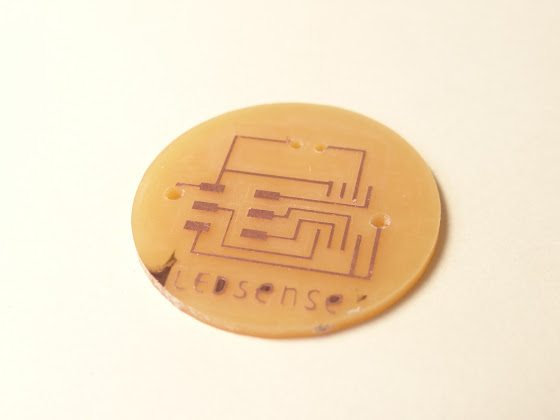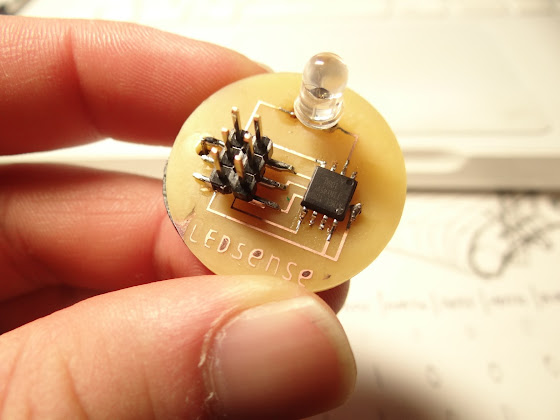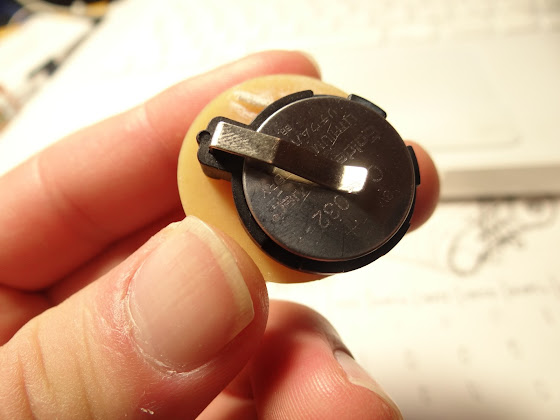# input devices

• Assignment
• This week, I made an "LED sensing board" which uses an LED as both a sensor and an actuator.
When an electric current runs through an LED, it glows.
Oppositely, when you apply a ray onto an LED, it generates electricity.
Using this function, this board let the LED turn on when it is dark and off when bright according to the value of brightness that the LED senses.

•• Firstly, I program ATmega 328P because a professor in my campus shares a program for 168, it was easy to rewrite it for 328P. (referring to this book)
• Here are the codes.
• ledsense328p.c
• ```#include <avr/io.h>
#include <avr/interrupt.h>
#include <avr/pgmspace.h>
#include <avr/wdt.h>
#include <util/delay.h>
#define F_CPU 1.0E6     //1MHz

int main(void){
unsigned char i;
int th;

th=0;
for(i=0;i<8;i++){		//8 times measurement
}

th=th>>3;		//average generated voltage

for(;;){
DDRC=0x20;
for(i=0;i<4;i++){
PORTC=0x20;
_delay_us(10);
PORTC=0x00;
_delay_us(10);
}
}
DDRC=0x20;
for(i=0;i<20;i++){
PORTC=0x00;
_delay_us(10);
}
}
DDRC=0;		//avoid digital output
PORTC=0;
}
}
```
• ledsense328p.c.make
• ```PROJECT=ledsense328p
SOURCES=\$(PROJECT).c
MMCU=atmega328p
F_CPU = 1000000

CFLAGS=-mmcu=\$(MMCU) -Wall -Os -DF_CPU=\$(F_CPU)

\$(PROJECT).hex: \$(PROJECT).out
avr-objcopy -O ihex \$(PROJECT).out \$(PROJECT).c.hex;\
avr-size --mcu=\$(MMCU) --format=avr \$(PROJECT).out

\$(PROJECT).out: \$(SOURCES)
avr-gcc \$(CFLAGS) -I./ -o \$(PROJECT).out \$(SOURCES)

program-bsd: \$(PROJECT).hex
avrdude -p m328p -c bsd -U flash:w:\$(PROJECT).c.hex

program-dasa: \$(PROJECT).hex
avrdude -p m328p -P /dev/ttyUSB0 -c dasa -U flash:w:\$(PROJECT).c.hex

program-avrisp2: \$(PROJECT).hex
avrdude -p m328p -P usb -c avrisp2 -U flash:w:\$(PROJECT).c.hex

program-avrisp2-fuses: \$(PROJECT).hex
avrdude -p m328p -P usb -c avrisp2 -U lfuse:w:0x7E:m

program-usbtiny: \$(PROJECT).hex
avrdude -p m328p -P usb -c usbtiny -U flash:w:\$(PROJECT).c.hex

program-usbtiny-fuses: \$(PROJECT).hex
avrdude -p m328p -P usb -c usbtiny -U lfuse:w:0x7E:m

program-dragon: \$(PROJECT).hex
avrdude -p m328p -P usb -c dragon_isp -U flash:w:\$(PROJECT).c.hex
```

## Mill a board with ATtiny45V

•••• Then I milled a board for ATtiny45V and rewrote the program but I couldn't make it.
Writing is always successful but an LED doesn't turn on.
• I tested another simple program on it and it was successful, the board itself doesn't have a problem.
So I found this is a program's problem but still cannot find how it is wrong.
• ledsense45.c
• ```#include <avr/io.h>
#include <avr/interrupt.h>
#include <avr/pgmspace.h>
#include <avr/wdt.h>
#include <util/delay.h>

int main(void){
unsigned char i;
int th;

th=0;
for(i=0;i<8;i++){		//8 times measurement
}
th=th>>3;		//average generated voltage

for(;;){
DDRB=0x08;
for(i=0;i<4;i++){
PORTB=0x08;
_delay_us(10);
PORTB=0x00;
_delay_us(10);
}
}
DDRB=0x08;
for(i=0;i<20;i++){
PORTB=0x00;
_delay_us(10);
}
}
DDRB=0;		//avoid digital output
PORTB=0;
}
}
```
• ledsense45.c.make
• ```PROJECT=ledsense45
SOURCES=\$(PROJECT).c
MMCU=attiny45
F_CPU = 1000000

CFLAGS=-mmcu=\$(MMCU) -Wall -Os -DF_CPU=\$(F_CPU)

\$(PROJECT).hex: \$(PROJECT).out
avr-objcopy -O ihex \$(PROJECT).out \$(PROJECT).c.hex;\
avr-size --mcu=\$(MMCU) --format=avr \$(PROJECT).out

\$(PROJECT).out: \$(SOURCES)
avr-gcc \$(CFLAGS) -I./ -o \$(PROJECT).out \$(SOURCES)

program-bsd: \$(PROJECT).hex
avrdude -p t45 -c bsd -U flash:w:\$(PROJECT).c.hex

program-dasa: \$(PROJECT).hex
avrdude -p t45 -P /dev/ttyUSB0 -c dasa -U flash:w:\$(PROJECT).c.hex

program-avrisp2: \$(PROJECT).hex
avrdude -p t45 -P usb -c avrisp2 -U flash:w:\$(PROJECT).c.hex

program-usbtiny: \$(PROJECT).hex
avrdude -p t45 -P usb -c usbtiny -U flash:w:\$(PROJECT).c.hex

program-dragon: \$(PROJECT).hex
avrdude -p t45 -P usb -c dragon_isp -U flash:w:\$(PROJECT).c.hex
```